•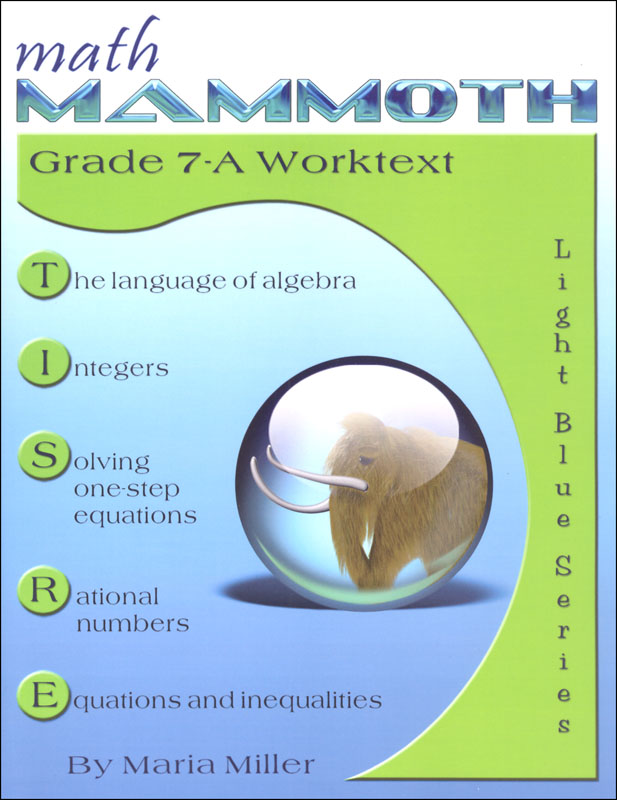•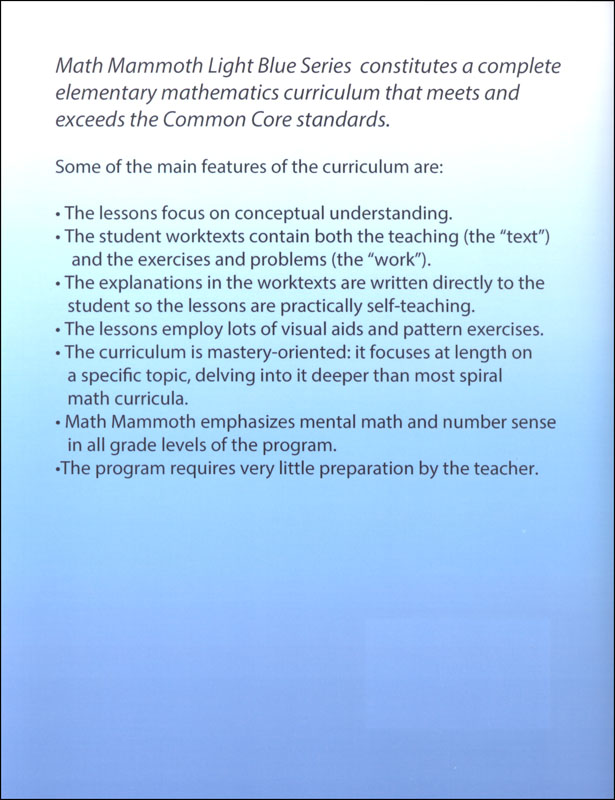•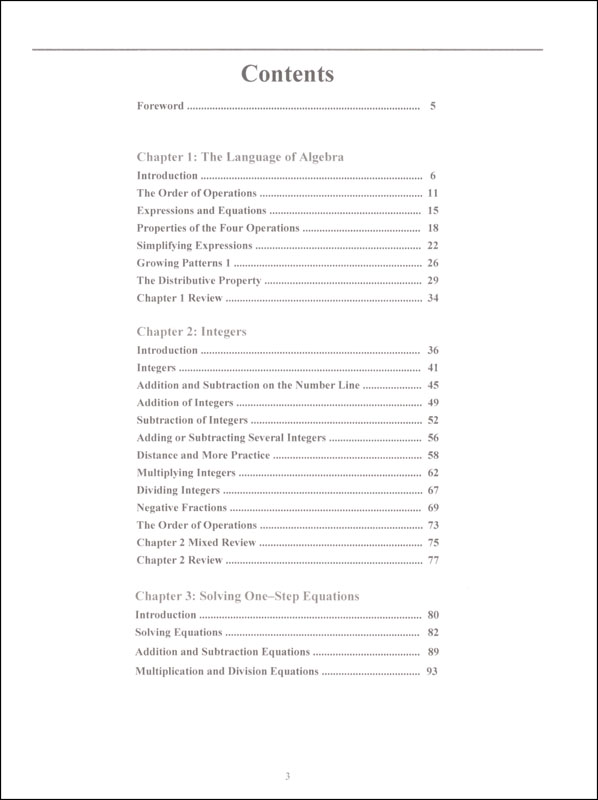•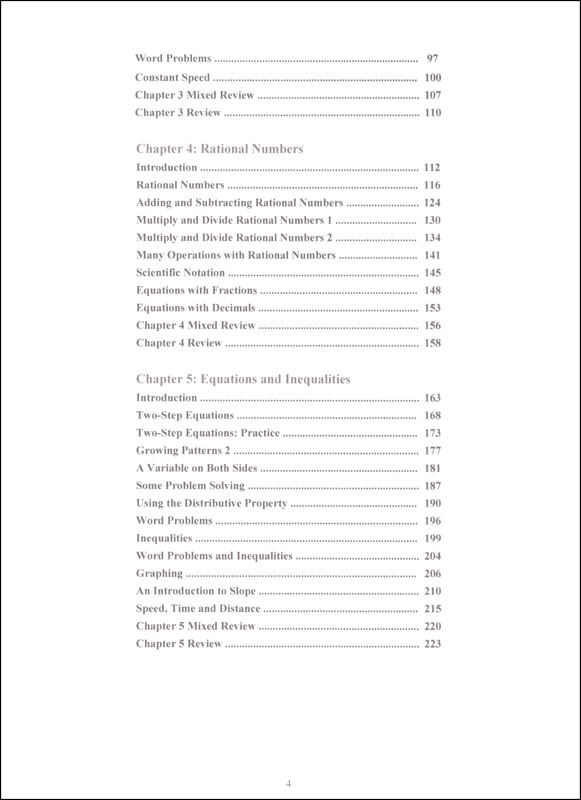•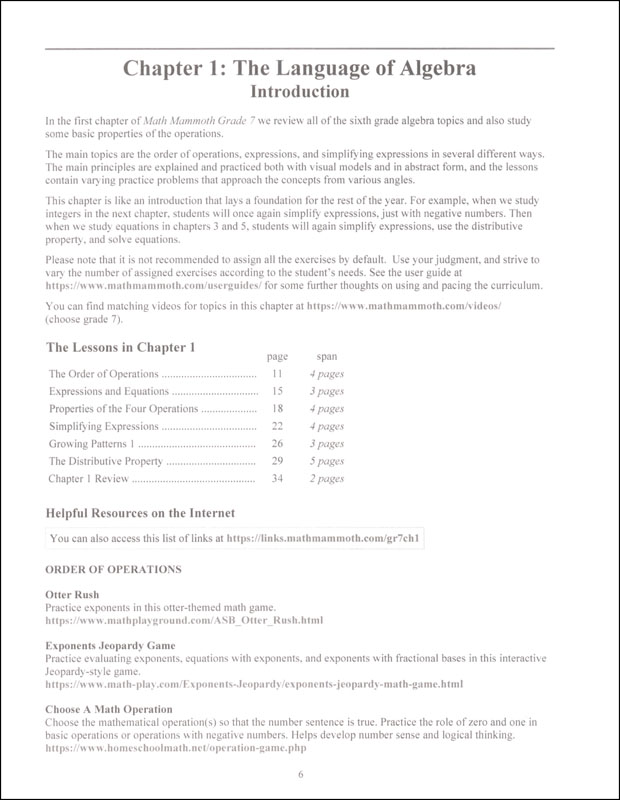•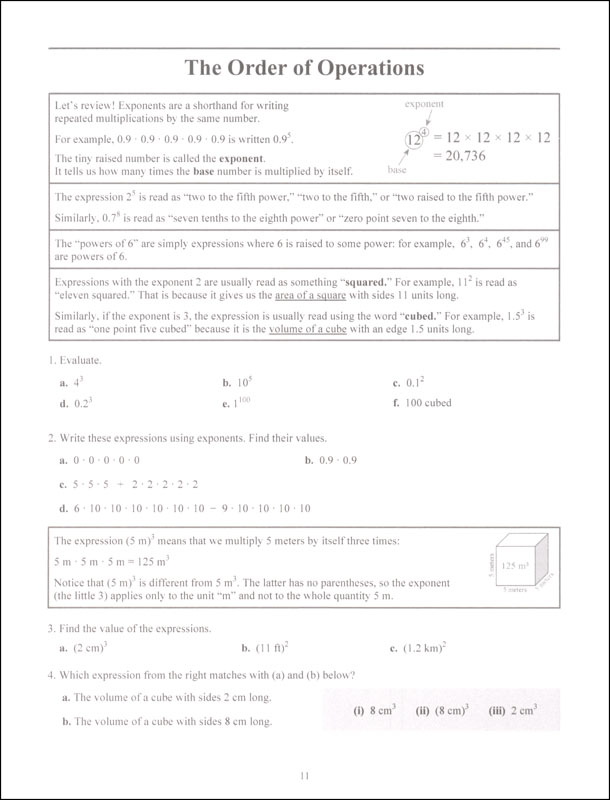•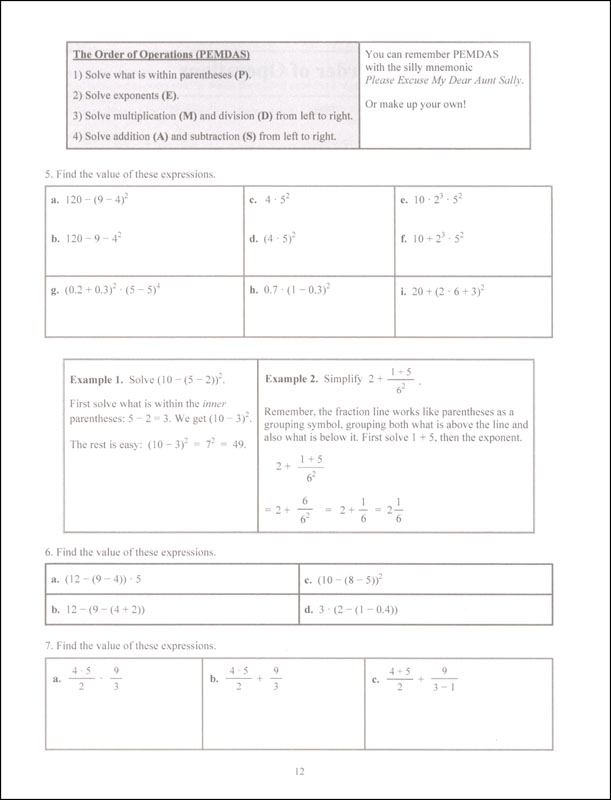•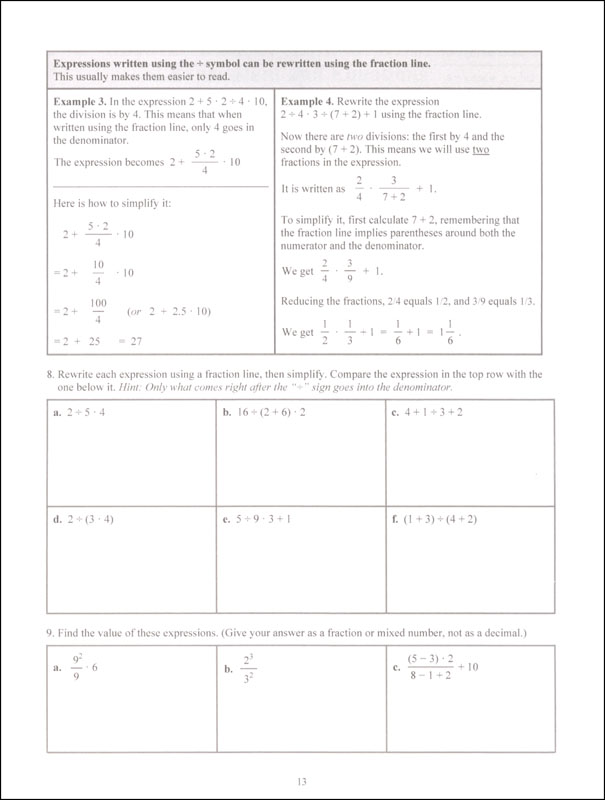•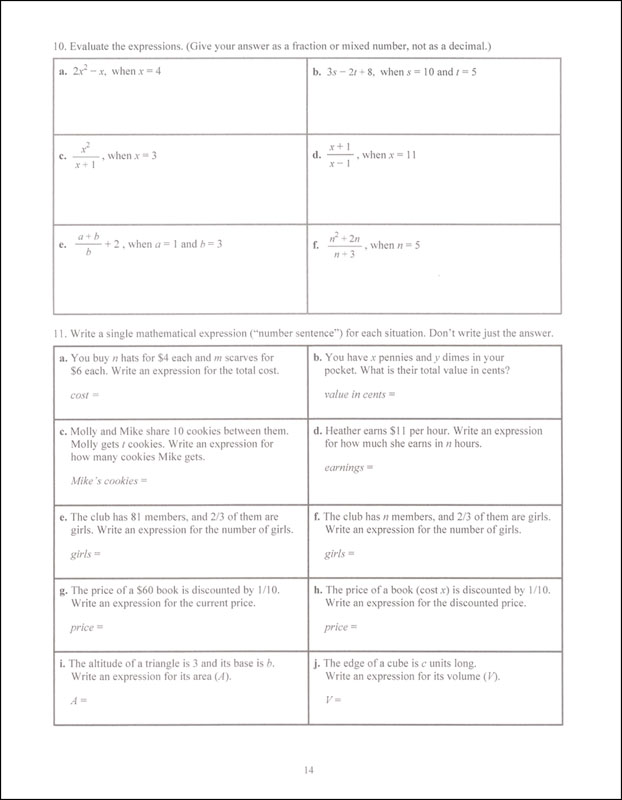# Math Mammoth Light Blue Series Grade 7-A Worktext

# 062261

Our Price: \$12.95
Retail: \$14.95
Save: 13.38% (\$2.00)
In Stock.
Qty:
Qty:

 Item #: 062261 9781954358027 7

#### Publisher Description:

The two books (worktext part A and part B) deal with

• introduction to basic algebra concepts
• integers and their operations
• solving one-step equations, including with negative numbers
• operations with negative rational numbers
• solving linear equations and writing equations for word problems
• graphing linear equations and an introduction to the concept of slope
• ratios, rates, proportions, and percent
• geometry: angle relationships, compass & ruler constructions, drawing problems, Pi and the area and circumference of a circle, cross-sections formed when cutting solids, surface area, and volume
• the Pythagorean theorem
• probability
• statistics

Math Mammoth Grade 7-A and Grade 7-B worktexts comprise a complete math curriculum for seventh grade mathematics studies. This is a pre-algebra course, and students can continue to an algebra 1 curriculum after studying it.

The curriculum meets the Common Core Standards (CCS) for grade 7 but also exceeds them in several places. For example, we study linear equations in more depth than required in grade 7 CCS, and the curriculum includes the Pythagorean Theorem, which in the CCS is in grade 8. You can access a document detailing the alignment information at this link or in the download version of this curriculum.

We start out with an introduction to basic algebra, which is in many ways a review of the same topics from 6th grade. The first chapter reviews the order of operations, the concepts of an expression and equation, and the distributive property. Students learn about the commutative and associative properties of addition and multiplication, and they simplify expressions that don't involve negative numbers.

In chapter 2, we study integers and their operations in detail. Some of this is, again, review from 6th grade, and some of it is new. The four operations of integers are explained with the help of two visual models: the number line and counters, hopefully providing an intuitive understanding of the processes. Students need to be able to add, subtract, multiply, and divide integers when they learn to solve equations in chapter 3. In the end of the chapter students also learn about negative fractions.

The lesson about distance contains a formula that may look unfamiliar to the teacher. You can find the distance between two integers by taking the absolute value of their difference. In symbols, the distance between a and b is | a − b | . The idea behind the formula is simple, though, and most people use that idea instinctively without knowing about the formula. For example, how far apart from each other are 14 and 92? To solve that mentally, we find their difference, but we take that difference in a positive sense. In other words, we do not calculate 14 − 92 = −78 and state that the distance is negative 78 units, but instead, we say the distance is 78 units because distance is always positive. The absolute value takes care of that: it turns any negative quantity into a positive one.

In the next chapter (chapter 3) students study simple one-step equations. They already know how the basics of how to solve these types of equations from 6th grade, but this time we use negative numbers in them.

Chapter 4 is titled Rational Numbers, which are simply fractions and certain decimals, so the student is already very familiar with them. The goal of the chapter is to be able to add, subtract, multiply, and divide both positive and negative fractions and decimals. We also solve simple equations involving fractions and decimals and learn about scientific notation and complex fractions.

The last chapter in part 7-A focuses on linear equations. The student learns to solve various types of linear equations and practices using those in simple word problems. We study linear inequalities but not to the same depth as linear equations. Lastly, the student graphs linear equations and is introduced to the concept of slope, which is the steepness of a line. The student will continue studying these topics with more details in an algebra 1 course.

Part B starts out with a study of ratios and proportions (chapter 6). Students study unit rates, proportions, proportional relationships and graphing, scaling geometric figures, floor plans, and maps.

The next chapter provides thorough lessons on the concept of percent. Students learn to solve a wide variety of problems involving percentages, including percentage of change, percentages of comparison, and simple interest problems.

Geometry is our focus in chapter 8. Students draw geometric figures using a a protractor and a ruler, and they also learn some basic geometric constructions. The other themes of this chapter are various angle relationships, area and the perimeter of a circle, conversions between units of area and of volume, surface area, volume, and cross-sections when solids are sliced with a plane.

Chapter 9 covers square roots, the Pythagorean Theorem, and its applications. I have included the Pythagorean Theorem in order to make the curriculum work as a pre-algebra course, and you can omit the entire chapter 9 if you are following the Common Core Standards for grade 7.

Chapter 10 is an introduction to probability. Besides learning the basic idea behind probability as the ratio of favorable events to all possible events, students compare experimental probabilities to theoretical ones in probability simulations and even design some on their own.

Lastly, in chapter 11, the curriculum covers statistical concepts. The major areas of study are random sampling and learning to compare two populations using some basic statistical measures and graphs.

Category Description for Math Mammoth:

Theres a whole lot to like about this well-conceived and inexpensive math program. The main features of this program, according to author Maria Miller, are:

• Focus is on conceptual understanding rather than rote problem-solving
• Teacher instructions are right in the book; no need to buy a separate teacher book
• Clear explanations are written for the student, so the lessons are virtually self-teaching
• Lessons use lots of visual and pattern exercises
• Mastery oriented:concentrates on each topic for a long time studying fewer topics per grade than with spiral
• Emphasis is on mental math and number sense
• Very little teacher preparation is required
• Content aligned to Common Core Standards

These are all valid claims and sum up this approach nicely. Its linear approach is similar to that of the MCP Math program. Topics are introduced, studied in depth, then the student proceeds to the next topic. Visually, it reminds me of Developmental Math because of the extensive use of pictorial representations. Users posting online have compared the course to Singapore Math, but I don't really see much similarity. While they both emphasize conceptual learning and mental computation skills, Math Mammoth has a much more traditional feel. A distinguishing feature of this program is the authors amazing ability to simplify and clarify math processes so children can understand them easily. Even in printed form, this course is the easiest on the budget using a strictly by-grade comparison. And for those purchasing the complete program on CD (or download), the savings are even greater. Imagine getting a complete, masterfully taught math curriculum for grades 1-7 for less than \$150. I would call that frugal and smart.

Each Grade Level Set consists of four components: Student Workbooks A & B, an (optional) set of Tests and Cumulative Reviews, and an Answer Key containing answers to both workbooks and tests. Currently, we carry the curriculum in print format or CD format by the grade level, Grades 1-3 and 4-7 combined on CD, and the entire course on CD. The course is also available as a download (go to mathmammoth.com site) for slightly less than the CD price. Both download and CD have colored student pages. All grade levels of printed materials are now available in your choice of black and white or color. Currently, the CDs also include bonus software, Soft-Pak - for Windows only (math, language arts and testing).

Student Workbooks A and B for each level have varying numbers of chapters, each focusing on a math topic. These are sometimes very broad. For example, one very large chapter (68 pages!) in Worktext 4-B is simply titled, “Division”. It reviews division, and then covers every facet of fourth grade division. Since division skills are being built step-by-step throughout the chapter, there’s really no need for review or testing until the end; but it does make for a long teaching unit. Each chapter begins with a short introduction. This is where you will find any teacher instruction for the chapter, including background information, strategies and ideas for teaching lesson concepts, a summary of lessons by title (including number of pages for each), and a list of Helpful Internet Resources for further exploration, practice and enrichment. If the chapter is on a skill introduced in a previous grade, the skill is reviewed before the new material is introduced. Lessons each include a complete explanation of each skill being taught, numerous examples and models (many visual) to help students understand the concepts behind the math, and a reasonable number of problems for students to work to ensure comprehension.

The lessons vary from 1-5 pages each. For planning, the author suggests simply dividing the number of pages in each book by the teaching days rather than planning to teach a lesson a day. This would generally mean covering 1-2 pages a day depending on grade level. After the last lesson in each chapter there are one or two reviews. Chapter tests are contained in the separate Tests and Cumulative Reviews Book, as are cumulative reviews (taken upon completion of each worktext chapter) and a comprehensive test for the grade level. A nice feature is the inclusion of grading instructions and scales. If remediation is indicated, the author provides a website (www. homeschoolmath.net) for additional math worksheets by grade level and skill. Worksheets are randomly generated within your provided parameters, so each is different.

Use the Skills Review Workbooks books alongside the Math Mammoth curriculum to review concepts that are being taught. Each book is divided into chapters that correspond with the curriculum chapters. These workbooks are optional, and are intended for those who want a spiral review. Each review in the workbook is one page in length and includes a variety of math exercises. Students might write math equations, color pictures, or solve puzzles to put their math skills to the test. Some children require more practice than others, so use only the review pages you need. Skills Review Workbooks are printed with color for a little more visual appeal, and the number of reviews vary from book to book. Answer Keys are a separate book and are also printed in color.

As mentioned briefly before, I find the author’s teaching methods very solid, efficient, and effective. Her goal is to imbue the student with an understanding of the concepts in math rather than just how to solve problems and this is evident in her instruction. She consistently shares strategies, “tricks” and revealing insights into the mysteries of math, essentially putting a teacher right on the page. An example is in teaching beginners to add numbers with sums greater than 10. She tells students that when adding 9 to a number, the 9 “really wants to be a ten”, so you should take one from the other number to make it a ten, and then add the rest. I have seen few other curriculums (see Miquon Math) that teach students alternate, flexible ways like this to approach operations. Another feature I liked in the book was using gridded workspaces for solving problems. Especially for students just starting to use multiple-digit operations, it’s helpful to get them in the habit of keeping answers neatly aligned in columns. I also like the scale problems in Grade 4’s multiplication and other chapters. These are much like the ones in CTP’s Balance Math program and very effective for understanding how equations work. There’s also a healthy balance of word problems in the lessons with realistic, practical contexts. The geometry sections are particularly good, too, with ample hands-on work. The author even includes printable manipulatives for constructing your own geometric solids.

Category Description for COMPREHENSIVE PROGRAMS - ELEMENTARY:

Primary Subject
Mathematics
7
ISBN
9781954358027
Author
Maria Miller
Format
Paperback
Brand Name
Taina Maria Miller
Weight
1.45 (lbs.)
Dimensions
11.0" x 8.5" x 0.56"
Browse 1 question Browse 1 question and 21 answers
Why did you choose this?
Rainbow Resource Center Store
Have used but wanted to not have to print for next year.
on May 30, 2021
We've used the 6th Grade program and it is superb!
on Jan 21, 2020
Have used but wanted to not have to print for next year.
on May 30, 2021
Have grade 6 and like the simplicity of it.
on Aug 14, 2020
Have grade 6 and like the simplicity of it.
on Aug 14, 2020
I was feeling lucky.
on Sep 19, 2019
5.0 / 5.0
2 Reviews
5 Stars
4 Stars
3 Stars
2 Stars
1 Star
2
0
0
0
0
Rated 5 out of 5
Great courses!
All of the Math Mammoth courses were great for my daughter who was never too enthused with math at all. I wish these had higher levels.
October 29, 2018
Purchased
over 4 years ago
Rated 5 out of 5
Excellent for pre-algebra study.
Good preparation material. Very well thought of curriculum.
August 10, 2018
Purchased
over 3 years ago

help desk software